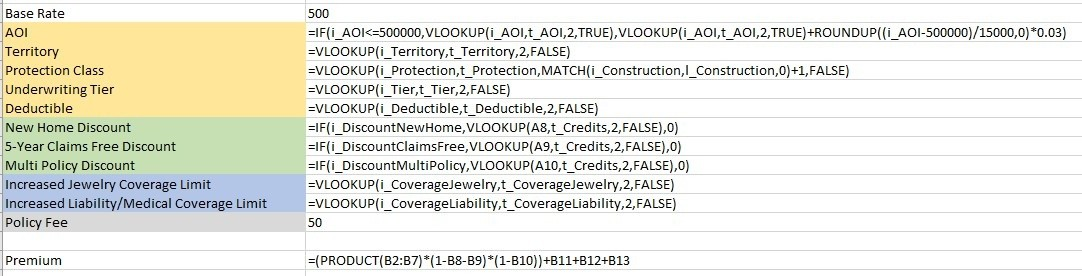Most actuaries are experts in Excel and love the tool. Actuarial model one of the most common usages of the software, since it makes it a breeze to build complex mathematical models and test them under various conditions.

In this article, I will walk you through building a simple actuarial rating model for homeowners’ insurance. I will implement the rating algorithm described in Basic Ratemaking.

## Use Defined Names in your Actuarial Models

Always use Defined Names in your actuarial model: name all your inputs, outputs, and data tables. Come up with a consistent naming convention, since this will make it easier to maintain your models going forward. Make sure your naming convention is sufficiently distinct.

Here is my preferred name convention:

• User Inputs:
• Convention: i_Name
• All user input names are prefixed with i_.
• Calculated Outputs:
• Convention: o_Name
• All outputs of the model are prefixed with o_.
• These are typically formula-driven cells.
• I typically exclude intermediate formulas from this definition.
• Tables:
• Convention: t_Name
• These are typically the lookup tables used in the model they are periodically updated by the actuaries.
• Lists:
• Convention: l_Name
• These are the lists that drive the input selections in the user interface. For example, the list of deductibles falls under this category.

The image below shows the names used in this sample actuarial model.## Data Tables

Combine all data tables into segregated worksheet(s) in your actuarial model. These are typically the tables containing various factors that are commonly updated by actuaries. They are used in lookup formulas to retrieve matching factors or rates, based on the user’s selections from the user interface page.

Also, make sure to separate tables by categories. In the homeowner’s rater, I separated these by Rate Relativity, Credits, and Additional Optional Coverages. Of course, you may have different categories depending on your model, but I suggest defining a color-coded categorization, as it will make future maintenance easier.

Always separate tables horizontally across your worksheet. Do not put tables below one another, as this will make it harder to maintain.Typically, data tables can also drive options for some of the inputs in the user interface. For example, the options available in the Deductible input are driven by the first column in the Deductibles table. So, it is important to define those names (l_Name) directly in this table. As the table is updated, the list options will automatically be updated in the user interface.

## User Interface

The next step is to design the user interface. It is important to decouple the user interface from the rest of the actuarial model.

Likewise, it is also important to validate your inputs. All list-driven inputs should be created using the List type validation in Excel, which will turn these fields into dropdowns.

You can also set the minimum and maximum value limitations to your other inputs. In this case, the Amount of Insurance input is being restricted to a specific range.The calculated results that will be displayed to the end user should also be referenced in the User Interface worksheet. In this example, the Premium is the only output that is meant to be in the user interface.

It is important to note that all inputs and outputs should be named as described above.

## Calculations

I recommend separating your formulas from the User Interface and Data Table sections. Depending on the complexity of your algorithm, it may be a good idea to have multiple calculation worksheets. In this actuarial model, we can easily combine all formulas into a single worksheet named Calculations.

Most of the formulas are simple VLOOKUPs, which will find the corresponding factor from a Data Table worksheet based on a given user input. For example, the VLOOKUP(i_Deductible,t_Deductible,2,FALSE) formula pulls the rate relativity from table t_Deductible for the input i_Deductible.

Some of the lookup formulas can be more complex, such as Discount rates. These not only use a VLOOKUP formula to pull the rate from the table but also apply that rate only if the user qualifies for it. The formula IF(i_DiscountNewHome,VLOOKUP(A8,t_Credits,2,FALSE),0) takes care of the lookup and the IF condition in the same cell.

The most complex formula in this actuarial model is used to calculate the AOI rate relativity. The data table only goes up to \$500,000. Beyond that, the rule is such that any additional \$15,000 value adds a factor of 0.03. So, the second part of the IF statement calculates the rate relativity if AOI is larger than \$500,000:

IF(i_AOI<=500000,VLOOKUP(i_AOI,t_AOI,2,TRUE),VLOOKUP(i_AOI,t_AOI,2,TRUE)+ROUNDUP((i_AOI-500000)/15000,0)*0.03)Finally, the Premium is calculated by multiplying the base rate of \$500 by all of the rate relativity factors using the PRODUCT formula: PRODUCT(B2:B7). This is subsequently multiplied by the applicable discount rates using formula (1-B8-B9)*(1-B10). Finally, the optional coverage fees are added, along with the policy fee of \$50, via formula B11+B12+B13.

I also published this workbook as a web application using SpreadsheetWeb.